﻿ Calculating Numbers For Income Tax Return With Pen, Glasses And Calculator. Editorial Stock Image - Image of deadline, income: 107759934

# Calculating numbers for income tax return with pen, glasses and calculator. Deadline, accounting.

## Calculating numbers for income tax return with pen, glasses and calculator

More similar stock imagesCalculating numbers for income tax return with pen.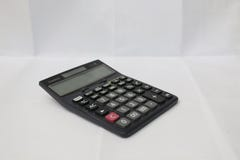A black CalculatorTablet and smart phones used in business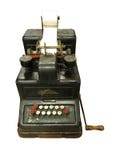Outline photo of old black calculatorCalculating numbers for income tax return.Tax returnStock Exchange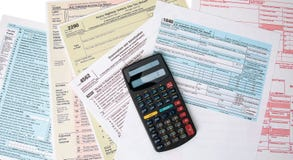Calculating Taxes FormsTax return form UK 2018
More stock photos from Maxopphoto's portfolio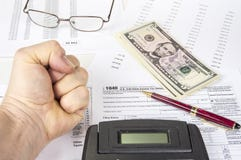Calculating numbers for income tax return with pen, glasses and calculator.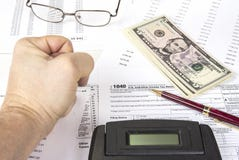Calculating numbers for income tax return with pen, glasses and calculator.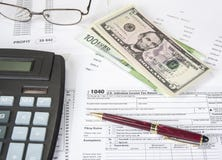Calculating numbers for income tax return with pen, glasses and calculator.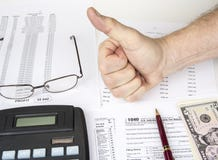Calculating numbers for income tax return with pen, glasses and calculator.
Related categories